## Friday, October 30, 2009

### 95

95 = 5 x 19.

950 + 951 + 952 + 953 + 954 + 955 + 956 and 950 + 951 + 952 + 953 + . . . + 95522 are prime numbers. Note that 6 and 522 lie between twin prime pairs.

The first 95 digits of 9493 form a prime.

95 is a number that cannot be written as a sum of three squares.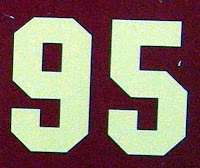I-95 is the longest, major U.S. north-south interstate highway, connecting Houlton, Maine, to Miami, Fla., and extending 1894 miles.

## Thursday, October 29, 2009

### 1927

1927 = 41 x 47.

1927 is the smallest number representable as the sum of three triangular numbers in 36 ways.

1927 = 211 – 112.

1927 is a number that cannot be written as the sum of three squares.

1927 is the sum of two pentagonal numbers in exactly two different ways.Yutaka Taniyama, whose conjecture was central to the proof of Fermat's Last Theorem by Andrew Wiles, was born in 1927 and died in 1958.

Source:
Prime Curios!

## Wednesday, October 28, 2009

### 1949

1949 is a prime number.

1949 has a representation as the sum of two squares: 1949 = 102 + 432 .

1949 is the hypotenuse of a primitive Pythagorean triple: 19492 = 8602 + 17492 .

1949 is 5453 is base 7 and 3635 in base 8.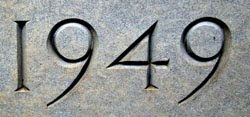East Germany was established officially as the German Democratic Republic in the year of
1949.

Source:
Prime Curios!

## Tuesday, October 27, 2009

### 2000

2000 = 24 x 53

2000 has two representations as the sum of two squares: 2000 = 82 + 442 = 202 + 402.

2000 is 5555 in base 7.

Mathematician John B. Cosgrove of St. Patrick's College in Dublin, Ireland, discovered a
2000-digit prime (for the millennium).1 short ton is
2,000 pounds.

Source:
Numeropedia

## Monday, October 26, 2009

### 2357

2357 is a prime number.

2357 is the smallest prime that contains all of the prime digits.

2357 has a representation as a sum of two squares: 2357 = 262 + 412.

2357 is the hypotenuse of a primitive Pythagorean triple: 23572 = 10052 + 21322.

22 + 33 + 55 + 77 is prime.

2357 is the sum of consecutive primes in at least two ways: 773 + 787 + 797 = 2357 and 461 + 463 + 467 + 479 + 487 = 2357.

2357 is the smallest number whose square begins with 4 identical (prime) digits: 23572 = 5555449.2357 is the number of pies used in the filming of The Great Race, which has a famous pie-throwing scene.

Source:
Prime Curios!

## Friday, October 23, 2009

### 2501

2501 = 41 x 61.

2501 is the product of the only two primes (41 and 61) that can be the last two digits of a perfect fourth power. If you replace the 5 by a 4, you get a fourth power: 2401 = 74.

2501 is a pentagonal number, having the form n(3n – 1)/2. In this case, n = 41.

2501 has two representations as a sum of two squares: 2501 = 12 + 502 = 102 + 492.

2501 is the hypotenuse of two primitive Pythagorean triples: 25012 = 1002 + 24992 = 9802 + 23012.Project
2501, from the film and manga Ghost in the Shell, is an artificial intelligence program that develops sapience.

Source:
Prime Curios!

## Thursday, October 22, 2009

### 2991

2991 = 3 x 997.

2991 is the smallest number with two prime factors summing to 1000—with three digits the same as the previous number (2491) with prime factors summing to a power of ten: 2491 = 47 x 53.

2991 is a number that cannot be written as the sum of three squares.

2991 is a semiprime that is not the sum of three pentagonal numbers.Lego set 2991 features Pooh and the Honeybees.

## Wednesday, October 21, 2009

### 4253

4253 is a prime number.

4253 has a representation as the sum of two squares: 4253 = 382 + 532.

4253 is the hypotenuse of a primitive Pythagorean triple: 42532 = 13652 + 40282.

M(4253) is the smallest titanic Mersenne prime: A Mersenne prime has the form 2n – 1; in this case, n = 4253. It was discovered Nov. 3, 1961, by Alexander Hurwitz using an IBM 7090 computer. The number has 1,261 decimal digits, making it a titanic prime because it has more than 1,000 decimal digits.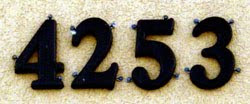The Massey Ferguson 4253 was a tractor produced between 1997 and 1999.

## Tuesday, October 20, 2009

### 5557

5557 is a prime number.

5557 is palindromic in base 2: 1010110110101.

5557 has a representation as the sum of two squares: 5557 = 92 + 742.

5557 is the hypotenuse of a primitive Pythagorean triple: 55572 = 13322 + 53952.

In summing primes, 2 + 3 + 5 + 7 + 11 + . . . + 3833 = 3847 + 3851 + . . . + 5557.NGC 5557 is a galaxy in the constellation Bootes.

Source: Prime Curios!

## Monday, October 19, 2009

### 8867

8867 is a prime number.

8867 is the least prime whose multiplicative persistence is a perfect number, 6. Multiply all the digits of a number n by each other, repeating with the product until a single digit is obtained. The number of steps required is known as the multiplicative persistence, and the final digit obtained is called the multiplicative digital root of n.

8867 is 105015 in base 6 and 34565 in base 7.

8867 is the lower prime of a difference of 20 between consecutive primes.The Magnificent Ambersons by Booth Tarkington is number 8867 in the Project Gutenberg catalog of online books.

Source: Wolfram MathWorld

## Friday, October 16, 2009

### 945

945 = 33 x 5 x 7.

945 is the first odd abundant number, discovered by Claude-Gaspar Bachet (1581-1638): 945 = 33 x 5 x 7 and its divisors sum to 975; 975 is greater than 945, so 945 is an abundant number. Odd abundant number are rare; only 23 occur below 10,000.

945 is the smallest odd primitive semiperfect number. A semiperfect number is equal to the sum of all or some of its proper divisors. A semiperfect number that is not divisible by any smaller semiperfect number is a primitive semiperfect number.

945 is 1661 in base 8.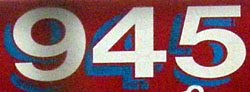NGC 945 is a galaxy in the constellation Cetus.

Source: Wolfram MathWorld

## Thursday, October 15, 2009

### 770

770 = 2 x 5 x 7 x 11.

770 is the number of digits of the 15th perfect number. A perfect number is a positive integer that is the sum of its proper divisors.

770 is 3322 in base 6 and 365 in base 15.

770 is a number with 16 divisors: 1, 2, 5, 7, 10, 11, 14, 22, 32, 55, 70, 77, 110, 154, 385, 770.The File 770 fanzine presents news of science fiction fandom. It is named for the party in Room 770 at the 1951 Worldcon in New Orleans that upstaged the convention.

## Wednesday, October 14, 2009

### 181

181 is a prime number.

181 can be represented as the sum of two squares: 92 + 102 = 181.

181 is the hypotenuse of a primitive Pythagorean triple: 1812 = 192 + 1802.181Rusty Romeos was the
181st short film starring the Three Stooges, released October 17, 1957.

Source:
Wikipedia

## Tuesday, October 13, 2009

### 2183

2183 = 37 x 59.

2183 is a number that cannot be written as a sum of three squares.

2183 is 2222212 in base 3.

2183 is a number n such that n2 + n + 2 is a palindrome: 21832 + 2183 + 2 = 4767674.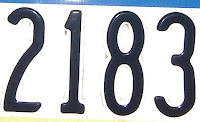NGC 2183 is a nebula in the constellation Monceros. This cloud of dust resides on the edge of the outer spiral arm of our galaxy.

Source:
Positive Integers

## Friday, October 9, 2009

### 886

886 = 2 x 443.

886 is 12021 in base 5.

886 is the smallest number representable as the sum of three triangular numbers in exactly 19 ways.

886 is the sum of six distinct positive pentatope numbers.886 is the telephone country code for Taiwan.

Source: Positive Integers

## Thursday, October 8, 2009

### 468

468 = 22 x 33 x 13.

468 is the smallest non-trivial number such that its own sum of digits is the same as its square's and its cube's sums of digits: 4 + 6 + 8 = 18; 2 + 1 + 9 + 0 + 2 + 4 = 18; 1 + 0 + 2 + 5 + 0 + 3 + 2 + 3 + 2 = 18.

468 is 3333 in base 5.

468 is the sum of ten consecutive primes: 29 + 31 + 37 + 41 + 43 + 47 + 53 + 59 + 61 + 67 = 468.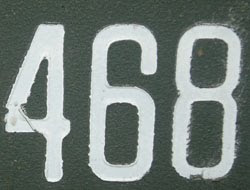NGC 468 is a galaxy in the constellation Pisces.

Source: Number Gossip

## Wednesday, October 7, 2009

### 544

544 = 25 x 17.

544 is 20200 in base 4.

544 is an Ulam number. The next Ulam number is uniquely the sum of two earlier distinct Ulam numbers.

544 has a representation as a sum of two squares: 544 = 122 + 202.544 Jetta is a minor planet orbiting the Sun.

Source: Wolfram MathWorld

## Tuesday, October 6, 2009

### 315

315 = 32 x 5 x 7.

315 = (4 + 3) × (4 + 1) × (4 + 5).

3152 = 253 + 263 + 273 + 283 + 293 = 99,225.

315 is a divisor of 642 - 1.

315 is a number divisible by each of its digits.315 is the telephone area code for Syracuse, New York.

## Monday, October 5, 2009

### 133

133 = 7 x 19.

133 has a unique representation as a sum of three squares: 133 = 42 + 62 + 92.

133 is 111 in base 11.

The reversal of
133 is prime (331). Note that 133.335 is the Dewey Decimal classification for numerology. If you reverse it, and add: 133.335 + 533.331, you'll discover the beast number, repeated!

133 is the smallest positive integer, n, for which the integer 10n + 5 is divisible by both 3 and 5 and for which none of the integers 10n + 1, 10n + 3, 10n + 7, and 10n + 9 are prime.133 is an octagonal number.It takes
133 years before the same phases of the moon occur on the same day of the year and also the same day of the week.

Source:
Prime Curios!

## Friday, October 2, 2009

### 701

701 is a prime number.

701, 7001, 70001, and 700001 are each prime.

2701 + 701 is prime.

701 = 54 + 43 + 32 + 21 + 10.

701 has a representation as a sum of two squares: 701 = 52 + 262.

701 is the hypotenuse of a primitive Pythagorean triple: 7012 = 2602 + 6512.The IBM 701 was the company's first production-line electronic digital computer, announced to the public in 1952.

Source: Prime Curios!

## Thursday, October 1, 2009

### 79

79 is a prime number.

79 is the smallest prime whose sum of digits is a fourth power: 7 + 9 = 16 = 24.

79 = 27 – 72.

79 is the maximum number of regions into which 12 lines divide a plane. It is possible to divide a flat pancake into 79 pieces by making 13 straight cuts.The chemical element gold has atomic number 79.

Source: Prime Curios!